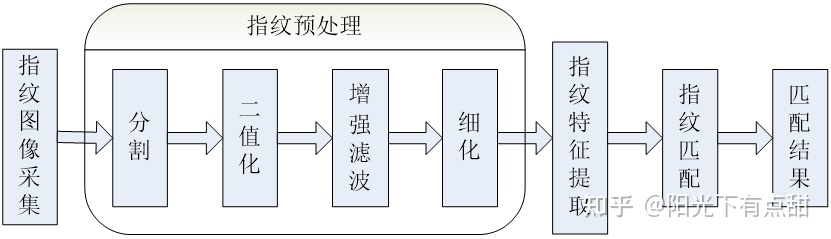# MATLAB指纹识别算法实现

191 篇文章 15 订阅

## 指纹识别概述

21世纪是信息化时代，在这个特殊的时代，我们的生活中电子设备越来越多，比如，笔记本电脑，ATM取款机，考勤系统，门禁系统和各种智能卡，网络中的网上银行，人人网账号等，都需要验证身份。对个人身份识别技术的要求不断提高，如果没有安全可靠和快捷的身份识别技术，电子商务、网上购物等就存在重大隐患。目前许多身份验证系统都采用“用户名+密码”的方式来进行用户访问控制，但此方法存在诸多隐患，比如密码被窃取、破解或遗忘。因此我们在与机器交互时急需一种准确、安全快捷的识别技术来取代现有的身份验证。

## 指纹识别的原理和方法

### 1.2.1 指纹的基本知识

（1）确定性：指纹脊线的轮廓和细节特征是在人的一生中基本上保持不变。自胚胎六个月到出生至死亡腐败之前，始终是没有很大变化的。指纹的确定性，还表现在它具有一定的复原性和难于毁灭的特性。

（2）唯一性：由于指纹脊线的连接关系千变万化，因此，即使两个不同的指纹有着相同的轮廓和相同数量的细节特征，它们的细节位置也是不可能完全相同的。

（3）可分类性：指纹可根据脊线和谷线的走向进行分类，一般可分为如图1-2所示的弓形、环形和螺旋形。

(a)弓形 (b) 环形 (c)螺旋形

（4）留痕性：指纹接触物体后会留下痕迹。指纹的这个特点，是与手掌表面附着面的污垢的性能紧密相关的。

### 1.2.2 指纹识别的原理及应用## Matlab在指纹识别中的应用

Matlab是一种高级的计算机语言, 具有独特的数学运算能力是matlab语言最突出的优点。许多在其他语言中描述起来很复杂的问题在matlab语言编程中却只需要一条专用的指令就可以完成。Matlab语言的所有计算都是基于矩阵的，所以matlab中的所有变量都被定义为矩阵, 它是一种解释型语言, 因此几乎没有语法格式上的限制。基于matlab实现指纹图像算法及仿真验证不仅有较高的准确率, 而且减小了仿真难度。

## 图像的二值化

### 2.2.3 静态阈值二值化

（1）人工设定阈值：该方案是根据实验或经验设置一个固定的阈值，然后对每个像素按照式来进行二值化处理。

（2）按照直方图确定阈值：利用原灰度图像分部的直方图来确定，设灰度值f取值是0~255之间的整数，f=0为黑色，f=255为白色。

## 指纹图像的滤波

（其中A>B>C>=0；P+2Q+2R=0）。

## 图像细化

### 2.4.2 改进的OPTA算法

)与图2-15所示的消除模板（八个）相比较， 若都不匹配，则P保留，否则将抽取出来的元素和图2-16的保留模板（六个）相比较，若与其中的一个匹配，则保留P，否则应该将P删除。重复利用以上的操作，将所有图像中的像素值进行比较直至不变为止。此算法是八连通的算法，基本都能够保证单像素的宽度。但该算法却不能使分叉点处彻底的细化，同时也会产生部分毛刺。

Matlab程序见附录A。细化结果图如图2-18：

（1）提取指纹的端点和交叉点

（2）去除图像边缘的端点

## 找出特征点

（1）single_point函数

（2）walk函数

（3）last1函数

single_point函数和walk函数都是找细化图像特征点的函数，因此可以设计另一个新的last1函数，通过执行

[pxy3,error2]=last1(thin,r,txy,num)

（1）脊线长度匹配

（2）三角形边长匹配

（3）点类型匹配

## 参考文献

 李俊山，李旭.数字图像处理.北京：清华大学出版社，

 阮秋琦.数字图像处理学.北京：电子工业出版社，

 祝恩，版建平等.自动指纹识别技术.长沙：国防科技大学出版社，

 查振元、朱华炳．电子门禁系统组成.机电产品开发与创新.2003，(2)：13—14

 胡士斌,杨卫平. 指纹图像复合分割算法研究[J]. 计算机工程与应用, 2006,40(12): 71273.

 李建华，马小妹，郭成安，基于方向图的动态闽值指纹图像二值化方法.大连理工大学学报.2002，42(5)：626-628

 家锋，唐降龙，赵泉.一个基于特征点匹配的联机指纹鉴别系统.哈尔滨工业大学学报，2002,34(1)：132-136

 刘文星，王肇圻，母国光．脊线跟踪及其在细化指纹后处理中的应用[J]．光电子,激光，2002，13(2)：184~187．

 王玮着，自动指纹识别系统关键技术研究，重庆，重庆大学光电工程学院，2007，80-82

 罗希平，田捷.自动指纹识别的图像增强和细节匹配算法.软件学报，2002-5，13(5)： 946-956

 姜腾云．指纹识别门禁系统的matlab仿真实现．江门：五邑大学，2011．

 乔治宏.基于细节结构的指纹特征提取及匹配算法研究.北京：北京工业大学硕士学位论文，2004-5.

附录A 预处理代码
function img = tuxiangyuchuli(path)
M=0;var=0;
[m,n,p]=size(I);
for x=1:m
for y=1:n
M=M+I(x,y);
end
end
M1=M/(m*n);
for x=1:m
for y=1:n
var=var+(I(x,y)-M1).^2;
end
end
var1=var/(m*n);
for x=1:m
for y=1:n
if I(x,y)>=M1
I(x,y)=150+sqrt(2000*(I(x,y)-M1)/var1);
else
I(x,y)=150-sqrt(2000*(M1-I(x,y))/var1);
end
end
end
figure, imshow(I(:,:,3)./max(max(I(:,:,3))));title(‘归一化’)
%************************************************************************

for x=1:H;
for y=1:L;
aveg=0;var=0;
for i=1:M;
for j=1:M;
aveg=I(i+(x-1)*M,j+(y-1)*M)+aveg;
end
end
aveg1(x,y)=aveg/(M*M);    %计算每一块的方差
for i=1:M;
for j=1:M;
var=(I(i+(x-1)*M,j+(y-1)*M)-aveg1(x,y)).^2+var;
end
end
var1(x,y)=var/(M*M);
end
end
Gmean=0;Vmean=0;
for x=1:H
for y=1:L
Gmean=Gmean+aveg1(x,y);
Vmean=Vmean+var1(x,y);
end
end
Gmean1=Gmean/(H*L);    %所有块的平均值
Vmean1=Vmean/(H*L);    %所有块的方差
gtemp=0;gtotle=0;vtotle=0;vtemp=0;
for x=1:H
for y=1:L
if Gmean1>aveg1(x,y)
gtemp=gtemp+1;
gtotle=gtotle+aveg1(x,y);
end
if Vmean1<var1(x,y)
vtemp=vtemp+1;
vtotle=vtotle+var1(x,y);
end
end
end
G1=gtotle/gtemp;V1=vtotle/vtemp;
gtemp1=0;gtotle1=0;vtotle1=0;vtemp1=0;
for x=1:H
for y=1:L
if G1<aveg1(x,y)
gtemp1=gtemp1-1;
gtotle1=gtotle1+aveg1(x,y);
end
if 0<var1(x,y)<V1
vtemp1=vtemp1+1;
vtotle1=vtotle1+var1(x,y);
end
end
end
G2=gtotle1/gtemp1;V2=vtotle1/vtemp1;
e=zeros(H,L);
for x=1:H
for y=1:L
if aveg1(x,y)>G2 && var1(x,y)<V2
e(x,y)=1;
end
if aveg1(x,y)< G1-100 && var1(x,y)< V2
e(x,y)=1;
end
end
end
for x=2:H-1
for y=2:L-1
if e(x,y)==1
if e(x-1,y) + e(x-1,y+1) +e(x,y+1) + e(x+1,y+1) + e(x+1,y) + e(x+1,y-1) + e(x,y-1) + e(x-1,y-1) <=4
e(x,y)=0;
end
end
end
end
Icc = ones(m,n);
for x=1:H
for y=1:L
if  e(x,y)==1
for i=1:M
for j=1:M
I(i+(x-1)*M,j+(y-1)*M)=G1;
Icc(i+(x-1)*M,j+(y-1)*M)=0;
end
end
end
end
end
figure, imshow(I(:,:,3)./max(max(I(:,:,3))));title('分割');
%************************************************************************
temp=(1/9)*[1 1 1;1 1 1;1 1 1];    % 模板系数、均值滤波
Im=double(I);
In=zeros(m,n);
for a=2:m-1;
for b=2:n-1;
In(a,b)=Im(a-1,b-1)*temp(1,1)+Im(a-1,b)*temp(1,2)+Im(a-1,b+1)*temp(1,3)+Im(a,b-1)*temp(2,1)+Im(a,b)*temp(2,2)+Im(a,b+1)*temp(2,3)+Im(a+1,b-1)*temp(3,1)+Im(a+1,b)*temp(3,2)+Im(a+1,b+1)*temp(3,3);
end
end
I=In;
Im=zeros(m,n);
for x=5:m-5;
for y=5:n-5;
sum1=I(x,y-4)+I(x,y-2)+I(x,y+2)+I(x,y+4);
sum2=I(x-2,y+4)+I(x-1,y+2)+I(x+1,y-2)+I(x+2,y-4);
sum3=I(x-2,y+2)+I(x-4,y+4)+I(x+2,y-2)+I(x+4,y-4);
sum4=I(x-2,y+1)+I(x-4,y+2)+I(x+2,y-1)+I(x+4,y-2);
sum5=I(x-2,y)+I(x-4,y)+I(x+2,y)+I(x+4,y);
sum6=I(x-4,y-2)+I(x-2,y-1)+I(x+2,y+1)+I(x+4,y+2);
sum7=I(x-4,y-4)+I(x-2,y-2)+I(x+2,y+2)+I(x+4,y+4);
sum8=I(x-2,y-4)+I(x-1,y-2)+I(x+1,y+2)+I(x+2,y+4);
sumi=[sum1,sum2,sum3,sum4,sum5,sum6,sum7,sum8];
summax=max(sumi);
summin=min(sumi);
summ=sum(sumi);
b=summ/8;
if (summax+summin+ 4*I(x,y))> (3*summ/8)
sumf = summin;
else
sumf =summax;
end
if   sumf > b
Im(x,y)=128;
else
Im(x,y)=255;
end
end
end
for i=1:m
for j =1:n
Icc(i,j)=Icc(i,j)*Im(i,j);
end
end
for i=1:m
for j =1:n
if (Icc(i,j)==128)
Icc(i,j)=0;
else
Icc(i,j)=1;
end;
end
end
figure,imshow(double(Icc));title('二值化');
%************************************************************************
u=Icc;
[m,n]=size(u)    %去空洞和毛刺
for x=2:m-1
for y=2:n-1
if u(x,y)==0
if u(x,y-1)+u(x-1,y)+u(x,y+1)+u(x+1,y)>=3
u(x,y)=1;
end
else u(x,y)=u(x,y);
end
end
end
figure,imshow(u)   %title('去毛刺')
for a=2:m-1
for b=2:n-1
if u(a,b)==1
if abs(u(a,b+1)-u(a-1,b+1))+abs(u(a-1,b+1)-u(a-1,b))+abs(u(a-1,b)-u(a-1,b-1))+abs(u(a-1,b-1)-u(a,b-1))+abs(u(a,b-1)-u(a+1,b-1))+abs(u(a+1,b-1)-u(a+1,b))+abs(u(a+1,b)-u(a+1,b+1))+abs(u(a+1,b+1)-u(a,b+1))~=1    %去空洞
if(u(a,b+1)+u(a-1,b+1)+u(a-1,b))*(u(a,b-1)+u(a+1,b-1)+u(a+1,b))+(u(a-1,b)+u(a-1,b-1)+u(a,b-1))*(u(a+1,b)+u(a+1,b+1)+u(a,b+1))==0     %去毛刺
u(a,b)=0;
end
end
end
end
end
figure,imshow(u)     %title('去空洞')
%*************************************************************************
v=~u;
se=strel('square',3);
fo=imopen(v,se);
v=imclose(fo,se);    %对图像开操作和闭操作
img=bwmorph(v,'thin',Inf);    %对图像进行细化
figure,imshow(img)
title('细化图')

function j = P (img, x, y, i)
switch (i)
case {1, 9}
j = img(x+1, y);
case 2
j = img(x + 1, y-1);
case 3
j = img(x, y - 1);
case 4
j = img(x - 1, y - 1);
case 5
j = img(x - 1, y);
case 6
j = img(x - 1, y + 1);
case 7
j = img(x, y + 1);
case 8
j = img(x + 1, y + 1);
end
%point函数
function txy=point(thin)
count = 1;
txy(count, :) = [0,0,0];
siz=min(size(thin,1),size(thin,2));
for x=40:siz - 40
for y=40:siz - 40
if (thin(y, x) )
CN = 0;
for i = 1:8
CN = CN + abs (P(thin, y, x, i) - P(thin, y, x, i + 1));
end
if (CN == 2)
txy(count, :) = [x, y,2];
count = count + 1;
end
if (CN == 6)
txy(count, :) = [x, y,6];
count = count + 1;
end
end
end
end
for i=1:count - 1
x(i) =txy(i, 1);
y(i)= txy(i, 2);
end
imshow(double(thin));
hold on;
plot(x,y,'.');
%cut函数
function txy=cut(thin,txy)
s(8,8)=0;
delta(8,8)=0;
n=size(txy,1);
for i=1:8
for j=1:8
mp{i,j}=thin(1+31*(i-1):31+31*(i-1),1+31*(j-1):31+31*(j-1));
s(i,j)=sum(sum(mp{i,j}))/(31*31);
mp{i,j}=(mp{i,j}-s(i,j)).^2;
delta(i,j)=sum(sum(mp{i,j}));
if delta(i,j)<=70
for k=1:n
if (txy(k,1)>=1+31*(i-1)&&txy(k,1)<=31+31*(i-1)&&txy(k,2)>=1+31*(j-1)&&txy(k,2)<=31+31*(j-1)&&txy(k,3)==2)
txy(k,:)=[0,0,0];
end
end
end
end
end
txy=txy(find(txy(:,1)),:);
plot(txy(:,1),txy(:,2),'ro');

%single_point函数
function [pxy2,error]=single_point(txy,r)
error=0;
x=txy(:,1);
y=txy(:,2);
n=length(x);
d(1:n,1:n)=0;
for j=1:n
for i=1:n
if (i~=j)
d(i,j)=sqrt((x(i)-x(j))^2+(y(i)-y(j))^2);
else
d(i,j)=2*r;
end
end
end
[a,b]=min(d);
c=find(a>r);
pxy2=txy(c,:);
pxy2=pxy2(find(pxy2(:,3)==2),:);
t=size(pxy2,1);
if t==0
error=1
else
plot(x,y,'b.');
hold on
plot(pxy2(:,1),pxy2(:,2),'r.');
end
%walk函数
function [error,a,b]=walk(thin,x0,y0,num)
error=0;
thin(y0,x0)=0;
t1=0;
for n=1:num
if error==1
break;
else
x=x0;
y=y0;
for x=x0-1:x0+1
if error==1
break;
else
for y=y0-1:y0+1
t1=sum(sum(thin(y0-1:y0+1,x0-1:x0+1)));
if (t1==0||t1>=2)
error=1;
a=x0;
b=y0;
break;
else
if (thin(y,x)==1&&(x-x0)^2+(y-y0)^2~=0)
if  (t1>=2 )
error=1;
break ;
else
thin(y,x)=0;
x0=x;
y0=y;
a=x0;
b=y0;
plot(x0,y0,'r.')
end
end
end
end
end
end
end
end
%last1函数
function [pxy3,error2]=last1(thin,r,txy,num)
error=0;
[pxy2,error]=single_point(txy,r);
n=size(pxy2,1);
l=1;
error2=0;
for i=1:n
[error,a,b]=walk(thin,pxy2(i,1),pxy2(i,2),num);
if error~=1
pxy3(l,1)= pxy2(i,1);
pxy3(l,2)= pxy2(i,2);
pxy3(l,3)= pxy2(i,3);
l=l+1;
error2=0;
plot(pxy2(i,1) ,pxy2(i,2),'r+');
end
end

%distance函数程序
function d=distance(x0,y0,num,thin)
num2=fix(num/5);
for i=1:num2
[error,a,b]=walk(thin,x0,y0,5*i);
if error~=1
d(i)=sqrt((a-x0)^2+(b-y0)^2);
else
break;
end
end
%find_point函数
function pxy=find_point(x0,y0,txy,num)
x=txy(:,1);
y=txy(:,2);
n=length(x);
l(1,n)=0;
lnn=1;
pxy(num,:)=[0,0,0];
for i=1:n
l(i)=sqrt((x(i)-x0)^2+(y(i)-y0)^2);
end
ll=sort(l);
for i=1:num
xiao=ll(i+lnn);
nn=find(l==xiao);
lnn=length(nn);
pxy(i,:)=[x(nn(1)),y(nn(1)),txy(nn(1),3)];
end
plot(x0,y0,'bo');
x0;
y0;
hold on
plot(pxy(:,1),pxy(:,2),'ro');
%主函数main
close all;
tic
clear;
thin1=tuxiangyuchuli('');
thin2=tuxiangyuchuli('');
figure;
txy1=point(thin1);
txy2=point(thin2);
[w1,txy1]=guanghua(thin1,txy1);
[w2,txy2]=guanghua(thin2,txy2);
thin1=w1;
thin2=w2;
txy1=cut(thin1,txy1);
txy2=cut(thin2,txy2);
[pxy31,error2]=last1(thin1,8,txy1,60)
[pxy32,error2]=last1(thin2,8,txy2,60)
error=1;
num=20;
cxy1=pxy31;
cxy2=pxy32;
d1=distance(cxy1(1,1),cxy1(1,2),num,thin1);
d2=distance(cxy2(1,1),cxy2(1,2),num,thin2);
f=(sum(abs((d1./d2)-1)));
if ff<=
error=0;
else
error=1;
end
c11=find_point(cxy1(1,1),cxy1(1,2),txy1,1);
c12=find_point(cxy1(1,1),cxy1(1,2),txy1,2);
c21=find_point(cxy2(1,1),cxy2(1,2),txy2,1);
c22=find_point(cxy2(1,1),cxy2(1,2),txy2,2);
cxy1(2,:)=c11;
cxy1(3,:)=c12(2,:);
cxy2(2,:)=c21;
cxy2(3,:)=c22(2,:);
x11=cxy1(1,1);  y11=cxy1(1,2);
x12=cxy1(2,1);  y12=cxy1(2,2);
x13=cxy1(3,1);  y13=cxy1(3,2);
x21=cxy2(1,1);  y21=cxy2(1,2);
x22=cxy2(2,1);  y22=cxy2(2,2);
x23=cxy2(3,1);  y23=cxy2(3,2);
dd1(1)=juli(x11,y11,x12,y12);
dd1(2)=juli(x12,y12,x13,y13);
dd1(3)=juli(x13,y13,x11,y11);
dd2(1)=juli(x21,y21,x22,y22);
dd2(2)=juli(x22,y22,x23,y23);
dd2(3)=juli(x23,y23,x21,y21);
ff=(sum(abs((dd1./dd2)-1)))
if ff<=1
error=0;
else
error=1;
end
cxy1(2:41,:)=find_point(pxy31(1,1),pxy31(1,2),txy1,40);
cxy2(2:41,:)=find_point(pxy32(1,1),pxy32(1,2),txy2,40);
f11=length(find(cxy1(:,3)==2));
f12=length(find(cxy1(:,3)==6));
f21=length(find(cxy2(:,3)==2));
f22=length(find(cxy2(:,3)==6));
fff=abs(f11-f21)/(f11+f12)
toc


08-2910904-14
01-05
11-03
03-30
12-17
04-14
08-282万+
12-301362
05-21
10-0129
03-1668
11-202202
03-1662
01-20
11-11873
07-271480点击重新获取扫码支付余额充值